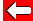# Factors

Another factor of 12, other than 2, 3 and 4 is 6, since:

12÷6=2

All numbers greater than 1 have at least two factors. The complete factors for 12 are:

1, 2, 3, 4, 6, 12.

Notice that the number itself is always a factor, as is 1. Numbers with only two factors are called primes.Go back a pageMaths MenuGo to next page